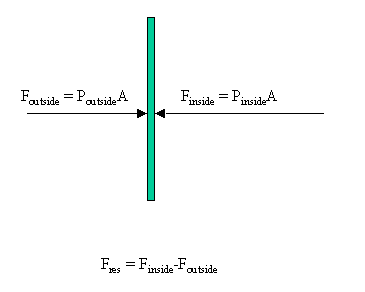# Solutions to SQA examination

### Paper I Solutions

```1. D		11. E			21. B
2. B		12. B			22. A
3. C		13. C			23. E
4. D		14. E			24. A
5. A		15. E			25. A
6. D		16. C			26. C
7. B		17. A			27. C
8. E		18. B			28. D
9. A		19. E			29. B
10.D		20. C			30. D

31.a.	VHor = Vcosq
VHor = 24.0cos60o
VHor = 12m/s

Note: the horizontal component of velocity is constant between P and X.

b.   SHor = VHort
SHor = 12x26
SHor = 312m

32.Fres = (9x104-4x104)3
Fres = 5x104x3
Fres = 15x104N	 (to the left)

33.a.	RP/RQ = RR/RS

When this is true the reading on the voltmeter is 0V.

b. 	The p.d. across the battery does not affect the above ratio of the
resistors. The bridge is therefore still balanced, and the voltmeter
reading will not change from 0V.

34.a.	VRMS = Vpeak/SQRT2
VRMS = 10/1.414
VRMS = 7.07V

b. 	f = 1/T

T = 8x0.2ms = 1.6ms
T = 1.6x10-3

f = 1/1.6x10-3
f = 625Hz

35.a.	The pupil assumes that there is 100% efficiency in the process
converting the energy stored in the capacitor into heat energy.
There is also the assumption that all the heat energy is absorbed
by the oil.

b. 	The energy stored in the capacitor can be calculated using: E = CV2/2

E = 5x10-6x102/2
E = 2.5x10-4J

This amount of energy would not produce a measurable change in the
temperature of the oil.

36.	For the first minimum:
Path difference = 1/2l
AB-AP = 1/2l
43-41 = 1/2l
2 = 1/2l
l = 4cm

b.	The fact that most of the radiation passes straight through indicates
that most of the atom must be empty space. However, the fact that some
"bounces back" provides evidence that the alpha particle occasionally
collides with a massive body. It is therefore reasonable to conclude
that atoms are mainly empty space with a large mass concentrated at
the centre.

END OF QUESTION PAPER